# ISEE Lower Level Quantitative : Quadrilaterals

## Example Questions

1 2 33 34 35 36 37 38 39 41 Next →

### Example Question #1 : How To Find The Area Of A Square

James found the area of the square below to be 36 centimeters squared.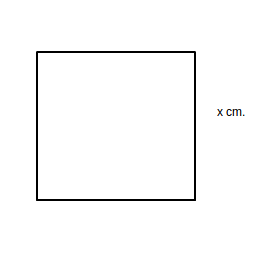What is the length of one side of the square?

Possible Answers:

9 centimeters

6 centimeters

36 centimeters

18 centimeters

8 centimeters

Correct answer:

6 centimeters

Explanation:

The area of a square can be found by multiplying the length of a side by itself.  36 is equal to 6 times 6, therefore the length of one side is 6 centimeters.

### Example Question #402 : Plane Geometry

Daphne found the area of the square below to be 81 centimeters squared.What is the length of one side of the square?

Possible Answers:

7 centimeters

9 centimeters

20 centimeters

8 centimeters

81 centimeters

Correct answer:

9 centimeters

Explanation:

The area of a square can be found by multiplying the length of a side by itself.  81 is equal to 9 times 9, therefore the length of one side is 9 centimeters.

### Example Question #403 : Plane Geometry

If the area of a square is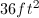, what is the length of each side?

Possible Answers: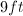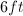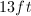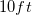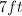Correct answer:Explanation:

To find the area of a square, the length must be multiplied by the height.  Since a square has four equal sides, the length and width will have the same measurement. We must, therefore, find the square root of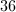.  Since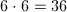,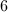is the square root of, which makes it the measurement for both the length and the width of the square.

### Example Question #404 : Plane Geometry

If the length of a rectangle is four times the width, and the width is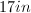, what is the perimeter of the rectangle?

Possible Answers: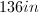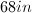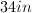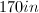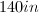Correct answer:Explanation:

In order to find the perimeter of a rectangle, we must add the length of all the sides together. In this case, however, we must first determine the length, which can be found by multiplying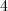by, because the length is four times the width, which had already been defined as.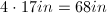Now we add together the four sides.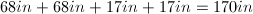### Example Question #405 : Plane Geometry

If the perimeter of a square is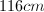, what is the length of each side?

Possible Answers: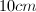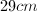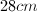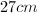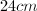Correct answer:Explanation:

To find the perimeter of a square, all the sides must be added together. Since a square hasequal sides, the length of each side can be determined by dividing the perimeter () by.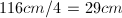### Example Question #631 : Geometry

The perimeter of a square is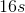. What is the length of one side?

Possible Answers: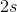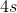Correct answer:Explanation:

To find perimeter, add up all the sides. Since this is a square, all of the sides are going to be the same. So, you can dividebyto find out what each side is. This gives an answer of### Example Question #407 : Plane Geometry

One side of a square ismeters long. What is the perimeter of the square?

Possible Answers: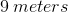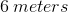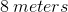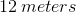Correct answer:Explanation:

The perimeter of a shape is the distance around the shape. The area is the space within the shape. To find the perimeter of a shape, we simply add all the sides together. Since all of a square's sides are equal, and a square has four sides, use the formula: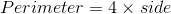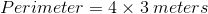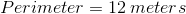### Example Question #408 : Plane Geometry

A right triangle has a base ofand a height of.

What is the perimeter of the rectangle made by combining 2 of these triangles along their hypotenuse?

Possible Answers:Correct answer:Explanation:

If the rectangle's length and width are 4 and 7, then perimeter, which is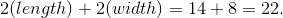### Example Question #409 : Plane Geometry

A square has a perimeter of, what is the lengthh of one side?

Possible Answers:Correct answer:Explanation:

The perimeter of a square is the sum of all of its sides. Since a square has four equal sides, the length of one side can be multiplied by four to find the perimeter.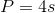In this problem, however, we are given the perimeter and must find the side.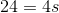Therefore, we should divide to find the missing side.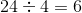1 2 33 34 35 36 37 38 39 41 Next →

### All ISEE Lower Level Quantitative Resources